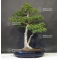##### The Japanese Bonsai specialist
Direct order Contact Help / Services Newsletter# Acer palmatum shishigashira ref:22060183

Acer palmatum shishigashiraref. : 9950

1.445,00

voluminous and/or heavy product extra shipping of 12,00

Available quantity : 1Order

###### Description

Height of the tree without its pot : 760 mm. Width of the branches : 660 mm.

Marine blue coloring enamelled pottery of : 540 x 410 x 112 mm. Sokan style.

Trunk’s diameter : 70 mm. Superb nebari of 260 x 130 mm.

Comes from aerial layering, perfectly formed bark, cultivated in pot for more than 20 years. Cutting wounds are currently healing on the back face. It will require about 2 years to heal completely. The current pot was deliberately chosen in order to enable the tree to heal easier. A less deep and smaller pot in 2 years will highlight this promising tree. Original bonsaï with its graceful silhouette and its good proportions. This tree presents a very beautiful roots’ set.

Shishigashira’s foliage is softly curly as the parsley’s leaves.

Foliage : bright green in spring becoming dark green during summer and red orange-toned yellow in autumn. Graceful and formed ramification.

Selected by Guy Maillot for the beauty its bark, its small size and its good proportions. This type of shishigashira with its styles and proportions become very rare because middle-sized trees are very wanted in Japan. Photographed in june 2018. Comes from master Takahashi’s nurseries.

#shishigashira 5.3 #tree 4.4 #proportions 4.1 #bonsai 3.6 #years 3.5 #trees 3.5 #very 3.4 #this 3.4 #palmatum 2.8 #graceful 2.8

Formule
(( ROUND((CHAR_LENGTH(b.article_nom)-CHAR_LENGTH(REPLACE(b.article_nom, 'shishigashira', '')))/LENGTH('shishigashira')) + ROUND((CHAR_LENGTH(b.article_description)-CHAR_LENGTH(REPLACE(b.article_description, 'shishigashira', '')))/LENGTH('shishigashira')) ) * 5.3) + (( ROUND((CHAR_LENGTH(b.article_nom)-CHAR_LENGTH(REPLACE(b.article_nom, 'tree', '')))/LENGTH('tree')) + ROUND((CHAR_LENGTH(b.article_description)-CHAR_LENGTH(REPLACE(b.article_description, 'tree', '')))/LENGTH('tree')) ) * 4.4) + (( ROUND((CHAR_LENGTH(b.article_nom)-CHAR_LENGTH(REPLACE(b.article_nom, 'proportions', '')))/LENGTH('proportions')) + ROUND((CHAR_LENGTH(b.article_description)-CHAR_LENGTH(REPLACE(b.article_description, 'proportions', '')))/LENGTH('proportions')) ) * 4.1) + (( ROUND((CHAR_LENGTH(b.article_nom)-CHAR_LENGTH(REPLACE(b.article_nom, 'years', '')))/LENGTH('years')) + ROUND((CHAR_LENGTH(b.article_description)-CHAR_LENGTH(REPLACE(b.article_description, 'years', '')))/LENGTH('years')) ) * 3.5) + (( ROUND((CHAR_LENGTH(b.article_nom)-CHAR_LENGTH(REPLACE(b.article_nom, 'this', '')))/LENGTH('this')) + ROUND((CHAR_LENGTH(b.article_description)-CHAR_LENGTH(REPLACE(b.article_description, 'this', '')))/LENGTH('this')) ) * 3.4) + (( ROUND((CHAR_LENGTH(b.article_nom)-CHAR_LENGTH(REPLACE(b.article_nom, 'very', '')))/LENGTH('very')) + ROUND((CHAR_LENGTH(b.article_description)-CHAR_LENGTH(REPLACE(b.article_description, 'very', '')))/LENGTH('very')) ) * 3.4) + (( ROUND((CHAR_LENGTH(b.article_nom)-CHAR_LENGTH(REPLACE(b.article_nom, 'palmatum', '')))/LENGTH('palmatum')) + ROUND((CHAR_LENGTH(b.article_description)-CHAR_LENGTH(REPLACE(b.article_description, 'palmatum', '')))/LENGTH('palmatum')) ) * 2.8) + (( ROUND((CHAR_LENGTH(b.article_nom)-CHAR_LENGTH(REPLACE(b.article_nom, 'graceful', '')))/LENGTH('graceful')) + ROUND((CHAR_LENGTH(b.article_description)-CHAR_LENGTH(REPLACE(b.article_description, 'graceful', '')))/LENGTH('graceful')) ) * 2.8) + (( ROUND((CHAR_LENGTH(b.article_nom)-CHAR_LENGTH(REPLACE(b.article_nom, 'foliage', '')))/LENGTH('foliage')) + ROUND((CHAR_LENGTH(b.article_description)-CHAR_LENGTH(REPLACE(b.article_description, 'foliage', '')))/LENGTH('foliage')) ) * 2.7) + (( ROUND((CHAR_LENGTH(b.article_nom)-CHAR_LENGTH(REPLACE(b.article_nom, 'formed', '')))/LENGTH('formed')) + ROUND((CHAR_LENGTH(b.article_description)-CHAR_LENGTH(REPLACE(b.article_description, 'formed', '')))/LENGTH('formed')) ) * 2.6)

## Secure payment## Delivery

Our logistic partners :